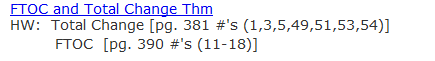•  2018 updated

1)   U substitution (Algebraic)   AP AB - Section 7.1 Notesheet
Homework Solutions: AP AB - Section 7.1 - WS Solutions

2)   U Substitution (Trigonometric)  AP AB - Section 7.2 Notesheet.pdf                 Section 7.2 WS Solutions

3)   U substitution (exponential and logarthmic)    AP AB Section 7.3 NS n WS KEY       Section 7.3 WS KEY

2017 not updated - If you need extra practice with U substitution, please look at the documents below.
2)   U substitution (exponential and logarthmic)   Notes
Homework Solutions:  Unit 5 WS 3a Solutions

4)   Additional Practice with U Substitiution
Homework Solutions WS 3B:  Unit 5 WS 3B U Substitution Solutions

4)   Evaluating Definite Integrals & Properties of Definite Integrals   Notes        solutions   WS #4 and page 371 Solutions
Homework: WS #4, Page 371/29-35
Page 401/ 37,38,40,43,57

5)   Separation of Variables (Differential Equations)   Notes
Homework Solutions: Hw Solutions

Topics to Review for Quiz   Unit 5 Quiz 1 Study Guide        Unit 5 WS 3 (U substitution) solutions

Additional problems for review:  Page 380/ 8,9,11,13,15,18,20,22,24,26,28

6)   Quiz      Monday

7)   Sigma (Summation) Notation   Notes      WS solutions

8)   Riemann Sums (L, R, M) for approximating area under a curve     page 359/ 1-4, 9,10       page 359 solutions

9)   Trapezoidal Rule for approximating area under a curve Notes     page 425 5 to 11 odds

RAM USING A TABLE OF VALUES

10)   Conceptual Theorems:   FTOC, , Total Change Theorem   Blank Fill in notes:   Notes and HWWS Solutions:   UNit 5 WS 6 FTOC Solutions

HW:  Total Change [pg. 381 #'s (1,3,5,49,51,53,54)]
FTOC  [pg. 390 #'s (11-18)]

HW Solutions   pg. 381 and 390
- See more at: http://cbsd.org//site/Default.aspx?PageID=9529&PageType=17&DomainID=1972&ModuleInstanceID=18460&EventDateID=101226&CurrentView=month#sthash.mPVeE1tJ.dpuf

11)   Conceptual Theorems: Average Value Theorem and MVT for integrals   Notes      MVT notes with Graphs

13)   Unit 5 Quiz 2   Free Response homework:   solutions

11)   Slope Fields   WS solutions   WS answers      FR solutions

12)  FREE RESPONSE KEY from problems assigned 3.13.2017 Unit 5 Free Response KEY McHugh

13)   Review   Unit 5 - Test Review 2017           Solutions

14)   Free Response   Unit 5 FR solutions.pdf

15)   Unit 5 Test